# 1.学习总结

## 1.1树结构思维导图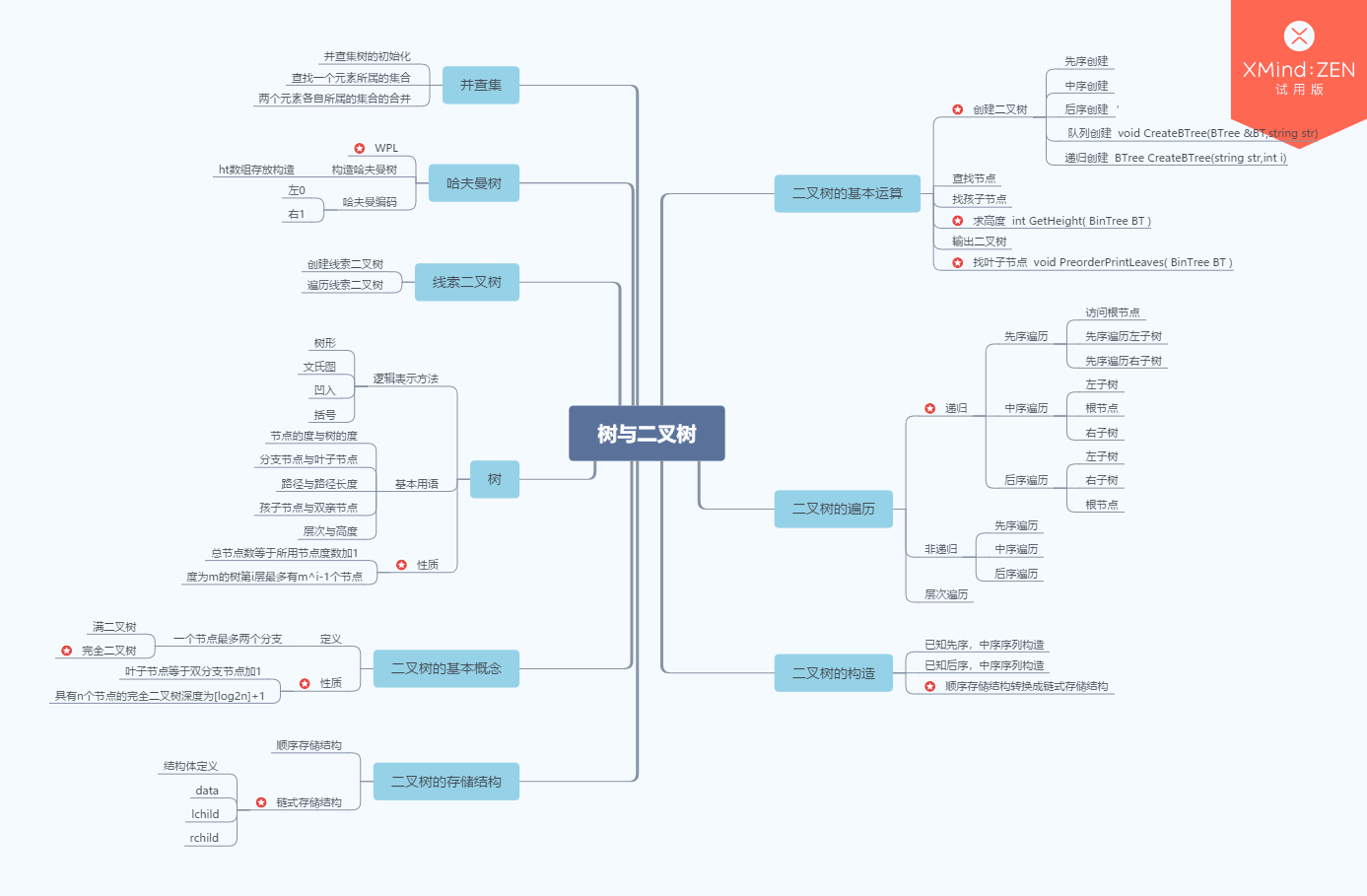## 1.2 树结构学习体会

• 1.学树结构觉得有点吃力了，因为有很多的内容，很零碎，还不能很好的联会贯通
• 2.难点重要点很多，二叉树，哈夫曼树等等
• 3.很多函数需要使用递归，很容易想不通掉进去
• 4.一些基本性质很重要，在写程序的时候有时用到会简化很多
• 5.知道了要写好伪代码，读懂伪代码的重要性

# 2.PTA实验作业

## 2.2 设计思路（伪代码或流程图）

 建二叉表达式树
void InitExpTree(BTree &T,string str) {

while(表达式未结束)
{
if(ch==操作数)
生成一个只有根节点的子树T。stacktree.push(T)
if(ch==运算符)
{
while(ch<op栈顶运算符)  栈顶优先级高，则
{        创建一个树结点T，数据为op.top()
stacktree弹出2个根节点T1，T2
T->lchild=T1,  T->rchild=T2
stacktree.push(T);
}
if(ch>op栈顶运算符)
op.push(ch)
if(ch==op栈顶运算符)  则 op.pop() 。只有(和)优先级相等
}
}
while(op.top()!='#')
{

s弹出2个根节点T1，T2
T->lchild=T1,  T->rchild=T2
s.push(T);
}
T=s.top();

计算表达式树
double EvaluateExTree(BTree T)
{

a=递归调用左孩子
b=递归调用右孩子

}


## 2.3 代码截图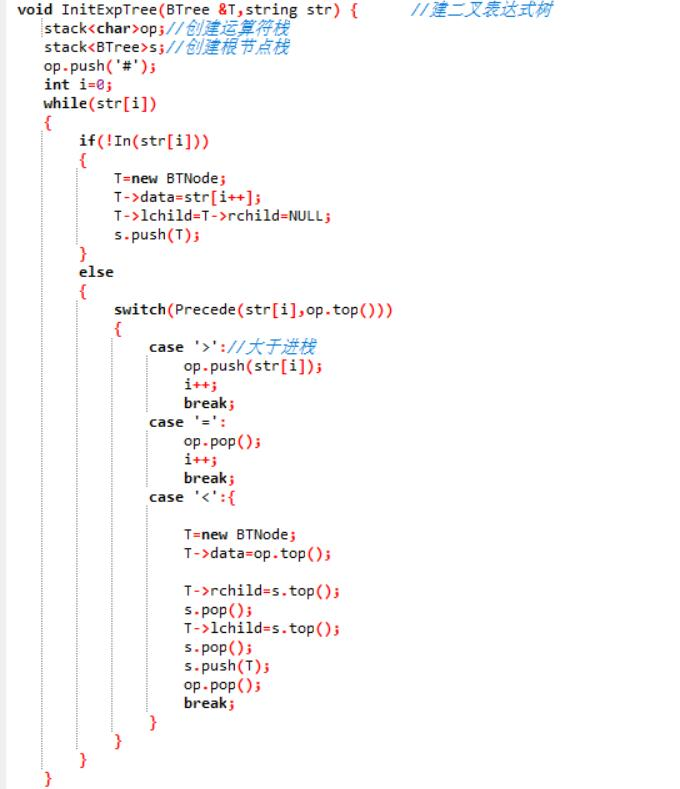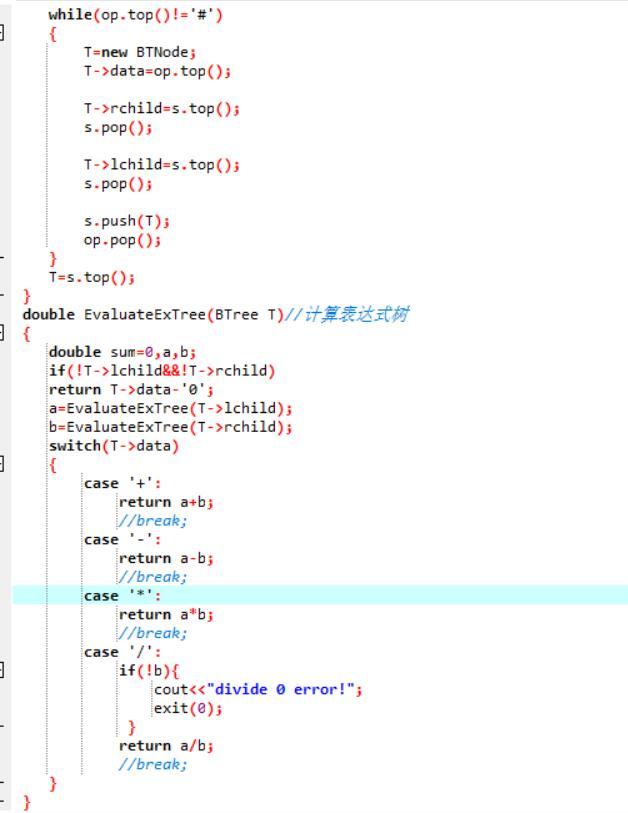## 2.4 PTA提交列表说明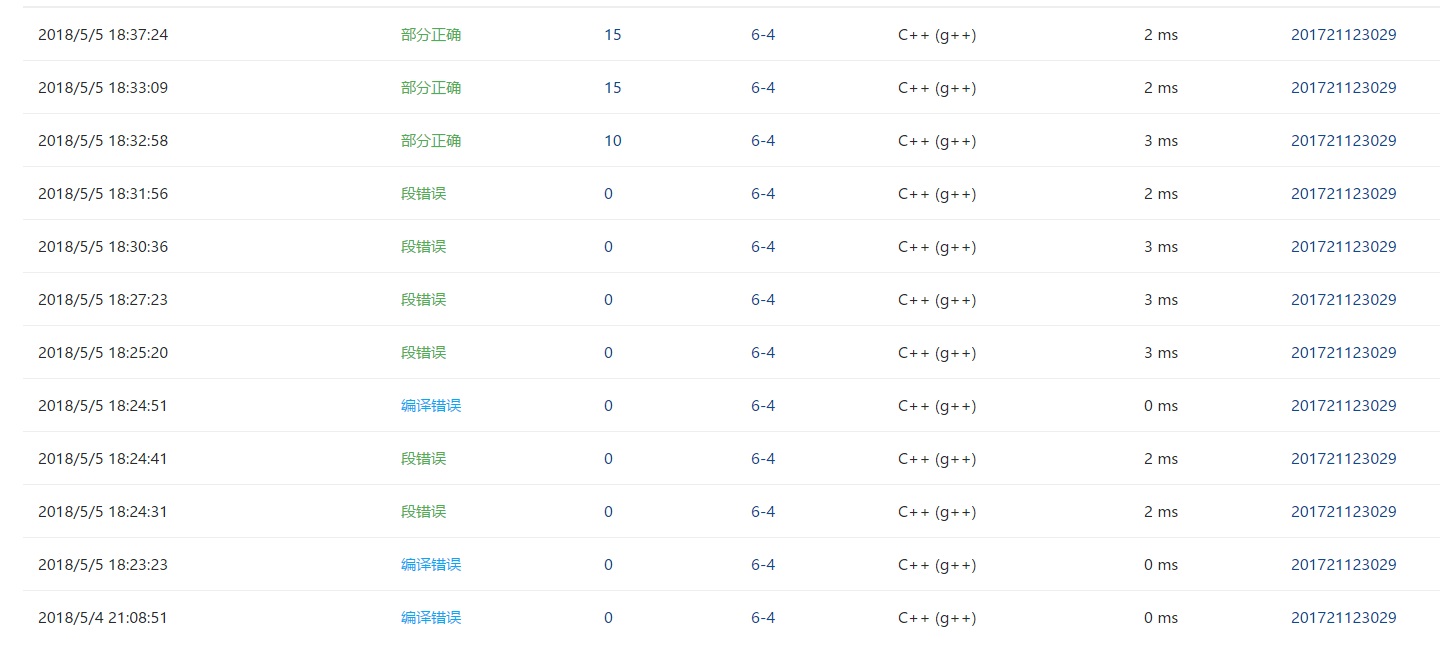## 2.2 设计思路（伪代码或流程图）

用递归法将二叉树的顺序存储结构转换成链式存储结构
trans（）

if（内容为#）表示该节点为空
else


找叶子节点并求WPL
leaves(BTree BT,int h)
if（节点不为空）{
该节点的左右孩子为空 sum=sum+(BT->data-'0')*h; sum为全局变量
递归判断左子树，并使高度加一
递归判断右子树，并使高度加一
}


## 2.3 代码截图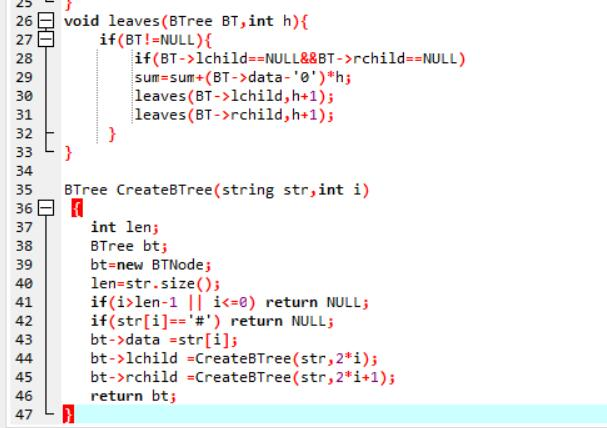## 2.4 PTA提交列表说明## 2.2 设计思路（伪代码或流程图）

构造哈夫曼树
CreateHT(HTNode ht[],int n)
{
n个叶子节点，哈夫曼树共有2n-1个节点
1.初始化哈夫曼数组ht，包含2n-1个节点

2.构造非叶子节点ht[i]（存放在ht[n]～ht[2n-2]中）

ht[lnode]和ht[rnode]的双亲节点置为ht[i]，并且ht[i].weight= ht[lnode].weight+ht[rnode].weight。
3.如此这样直到所有2n-1个非叶子节点处理完毕。
}

寻找非叶子节点
void notLeaves(HTNode ht[],int n)
{

if（左右孩子节点不为-1）

}


## 2.3 代码截图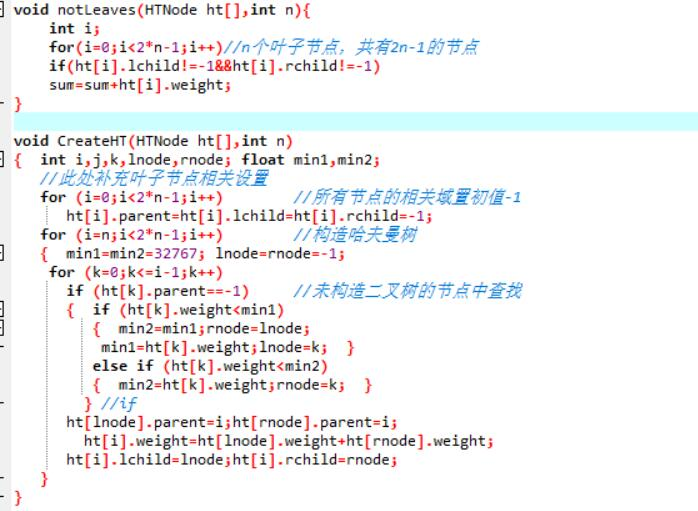## 2.4 PTA提交列表说明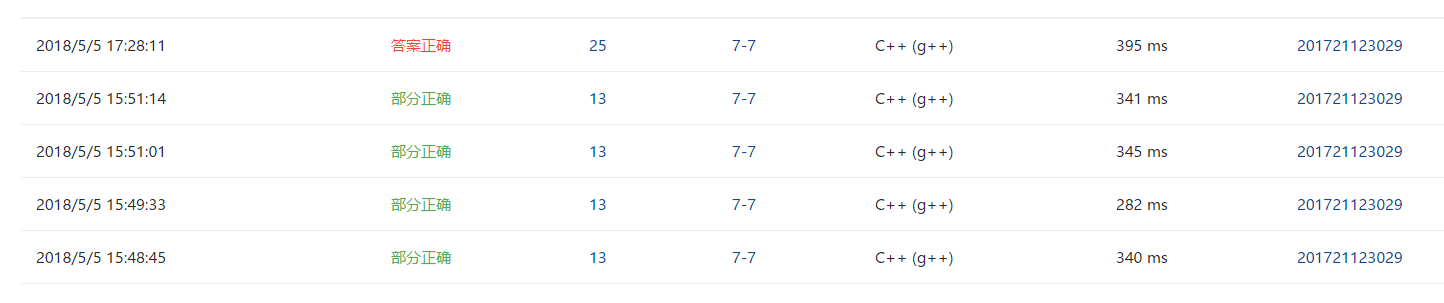# 3.截图本周题目集的PTA最后排名

## 3.1 PTA排名# 4. 阅读代码

• 题目：求二叉树的宽度
#include<iostream>
#include<queue>
#include<string>
#include<math.h>
using namespace std;
typedef struct node{
char data;
struct node *lchild;
struct node *rchild;
}BTNode;
typedef BTNode *BTree;
BTree CreateBTree(string str,int i);
int wide(BTree BT,string str);
int fun(BTree b);
void levelnumber(BTree b,int h,int a[]);
int main(){
int i=1,w;
BTree BT;
string a;
cin>>a;
BT=CreateBTree(a,i);
w=fun(BT);
cout<<w;
return 0;
}
BTree CreateBTree(string str,int i)
{
int len;
BTree bt;
bt=new BTNode;
len=str.size();
if(i>len-1 || i<=0) return NULL;
if(str[i]=='#') return NULL;
bt->data =str[i];
bt->lchild =CreateBTree(str,2*i);
bt->rchild =CreateBTree(str,2*i+1);
return bt;
}
void levelnumber(BTree b,int h,int a[])
{
if (b==NULL)   return;
else
{
a[h]++;
levelnumber(b->lchild,h+1,a);
levelnumber(b->rchild,h+1,a);
}
}
int fun(BTree b)
{
int width=0,i;
int a;
for (i=1;i<20;i++)   a[i]=0;		//a设置所有元素初始化为0
levelnumber(b,1,a);
i=1;
while (a[i]!=0)
{
if (a[i]>width)   width=a[i];
i++;
}
return width;
}


# 5. 代码Git提交记录截图posted @ 2018-05-05 19:00  yawlc  阅读(435)  评论(2编辑  收藏  举报